# Neural Style Transfer on Real Time Video (With Full implementable code)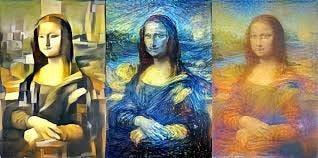Neural Style Transfer

Written: 29th May 2019 by Sourish Dey

In recent few years, we have experienced the application of computer vision in almost every nook and corner of our life — thanks to the availability of huge amounts of data and super-powered GPUs, which have made training and deployment of convolutional neural networks(CNN) super easy. In my previous article, I discussed one such value-added application in business. One of the most interesting discussions today around within machine learning is how it might impact and shape our cultural and artistic production in the next decades. Neural style transfer is one of the most creative applications of convolutional neural networks.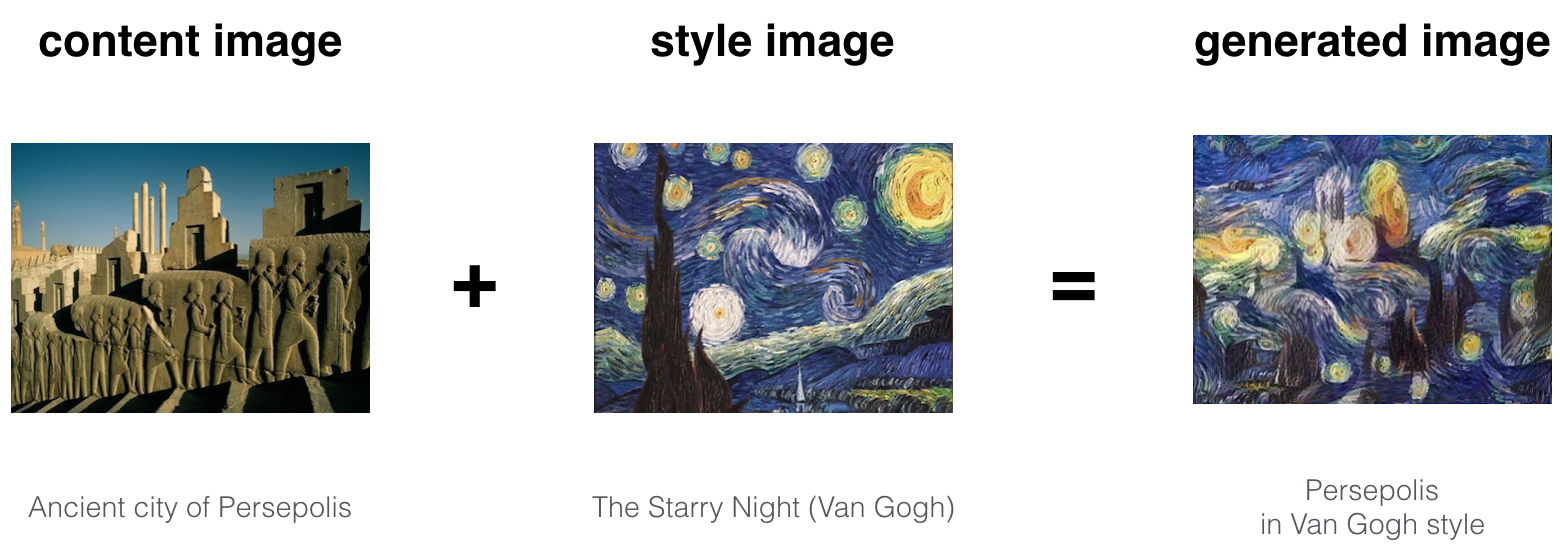By taking a content image and a style image, the neural network can recombine the content and the style image to effectively creating an artistic(recomposed) image. Although there are many apps like Prisma which generates artistic styles to pictures taken from mobile, in almost no time, the purpose of this article is to understand the science and art behind this seemingly difficult concept. The real-time implementable code is shared here.

### Background-Neural Style Transfer

Neural Style Transfer was concept was first brought on the seminal paper by Gatys, Ecker, and Bethge(A Neural Algorithm of Artistic Style in 2015) demonstrating a method for combining the artistic style of one image with the content of another image. The basic idea is to take the feature representations learned by a pre-trained deep convolutional neural network like VGG 16 (typically trained for image classification or object detection) to obtain separate representations for the style and content of an image. Once these representations are found, then we try to optimize a generated image to recombine the content and style of different target images. So the concept randomizes texture, contrast, and color while preserving shape and semantic features(central aspect) of content image. Although it is somewhat similar to a color transformation, it separates itself with the ability to transfer textures(styles) and other miscellaneous distortions that are impossible with classic color filters.

### Problem Statement- Isn’t it an optimization problem?

So the problem statement here is given a content photo X and style photo Y how can we transfer the style of Y to the content X to generate a new photo Z. How can we train CNN to process and optimize the differences(the difference between X and Y)to reach an optimum global(Z)?

Basic Overview- Neural Style Transfer(source)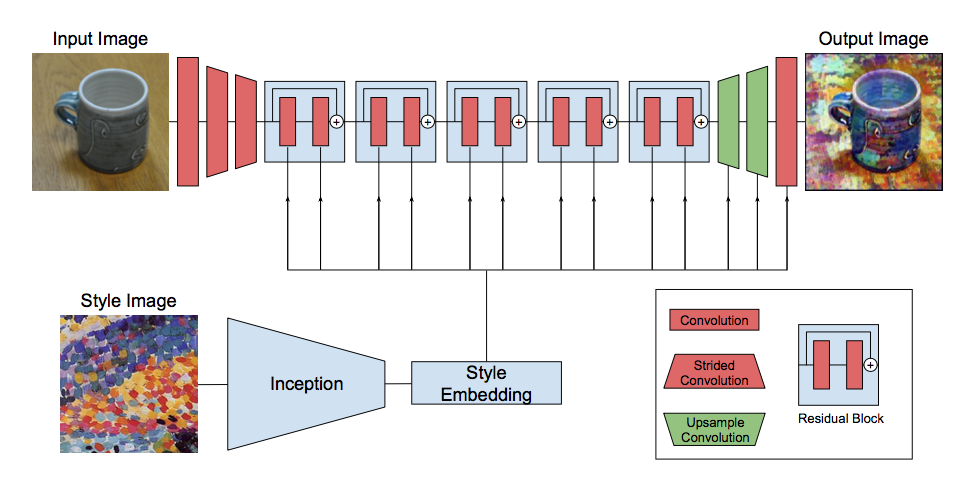Basic Overview- Neural Style Transfer(source)

### Optimization problem overview

Gatys shows in the original paper(A Neural Algorithm of Artistic Style in 2015)that “transferring style(textures)one image to another content image is posed as an optimization problem, which can be solved by training a deep neural network”.Following are the components of this puzzle:

• Content loss:It represents how similar the content of the style transfer network’s output image(styled image) is to the content of the input image, or “content target”,it tends to zero if the input image(X) and styled image(Z)similar to each other in terms of content and grows if they differ. To capture the content of the image properly we need to preserve the spatial(shape/boundaries etc.)/high level features of the content image. Since the image classification convolutional neural networks like VGG16 are forced to learn high-level features/abstract representations or the “content” of images at the deeper layer, for content comparison we use activation/feature map at some deeper layer(L)- 1 or 2 layers prior to the output(softmax) layer. The choice of L (which layer to extract the content features from) can be chosen as desired, and the deeper the chosen layer is, the more abstract the output image will look. So L is a hyperparameter of the network.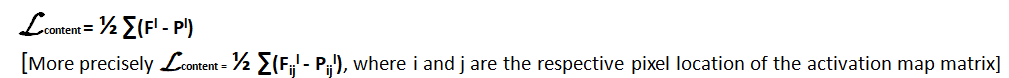• Gram matrix and Style loss: Although slightly more complex, the style loss between the original style image(Y) and the network’s output image(Z) is also computed as a distance between features (activation map) extracted from layer outputs from VGG-16. The main difference here is that instead of comparing feature representations directly from the layer activation matrix of VGG-16, those feature representations are transformed into spatial correlation matrix(within an activation map), which is done by calculating Gram matrix. The gram matrix contains the correlation between every pair of feature maps at layers for the style image. So essentially Gram matrix captures the tendency of features which co-occurs at different parts of the image.It represents the inner dot product of a set of vectors and this captures the similarity between two vectors, since if two vectors are similar to one another, then their dot product will be large, and thus the Gram matrix will be large.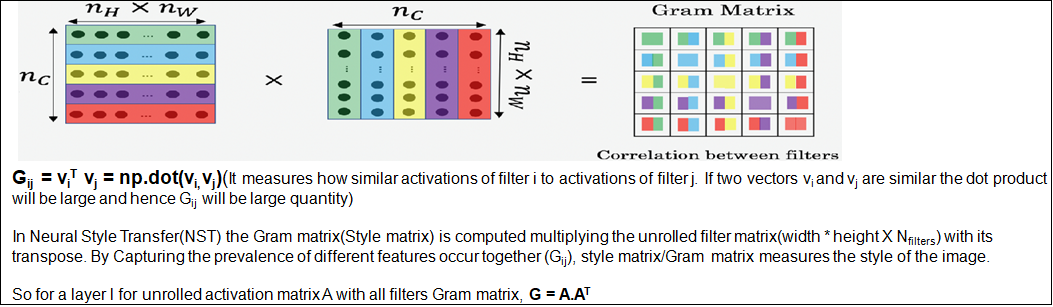Source

• In the original paper, Gatys suggested taking the combination of shallow and deep conv layers to calculate style loss for style representation. So style loss is the sum of mean squared difference(Euclidian distance) in style features between original style image(Y) and generated image(Z) for each conv layer activation matrix.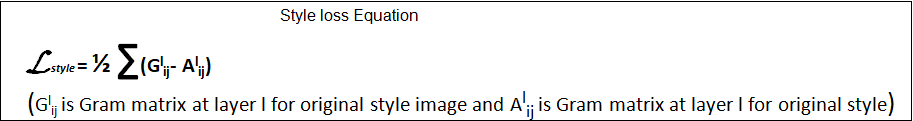• Overall loss: Overall loss is the weighted sum of the content loss and style loss as follows.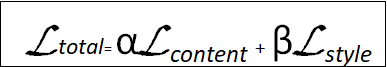• The network is trained to simultaneously minimize content loss and style loss. α and β are weights of content loss and style loss resp and are again hyperparameters of the overall CNN. Choices of these values solely depend on how much content or style needs to be kept in the generated image(Z). Here we start with a random(white noise) image matrix and in each iteration calculate the feature map distance(overall loss) between both content image(content loss) and style image(style loss) to calculate the overall loss. So this loss is back-propagated through the network and we need to minimize the total loss by suitable optimization technique(equivalent to Gradient Descent) over iterations(few thousands) to update the random image matrix as close as both content and style image. We continue the process until we get the minimum threshold loss value to generate the optimized blended image(Z), which looks similar to both content(X) and style image(Y).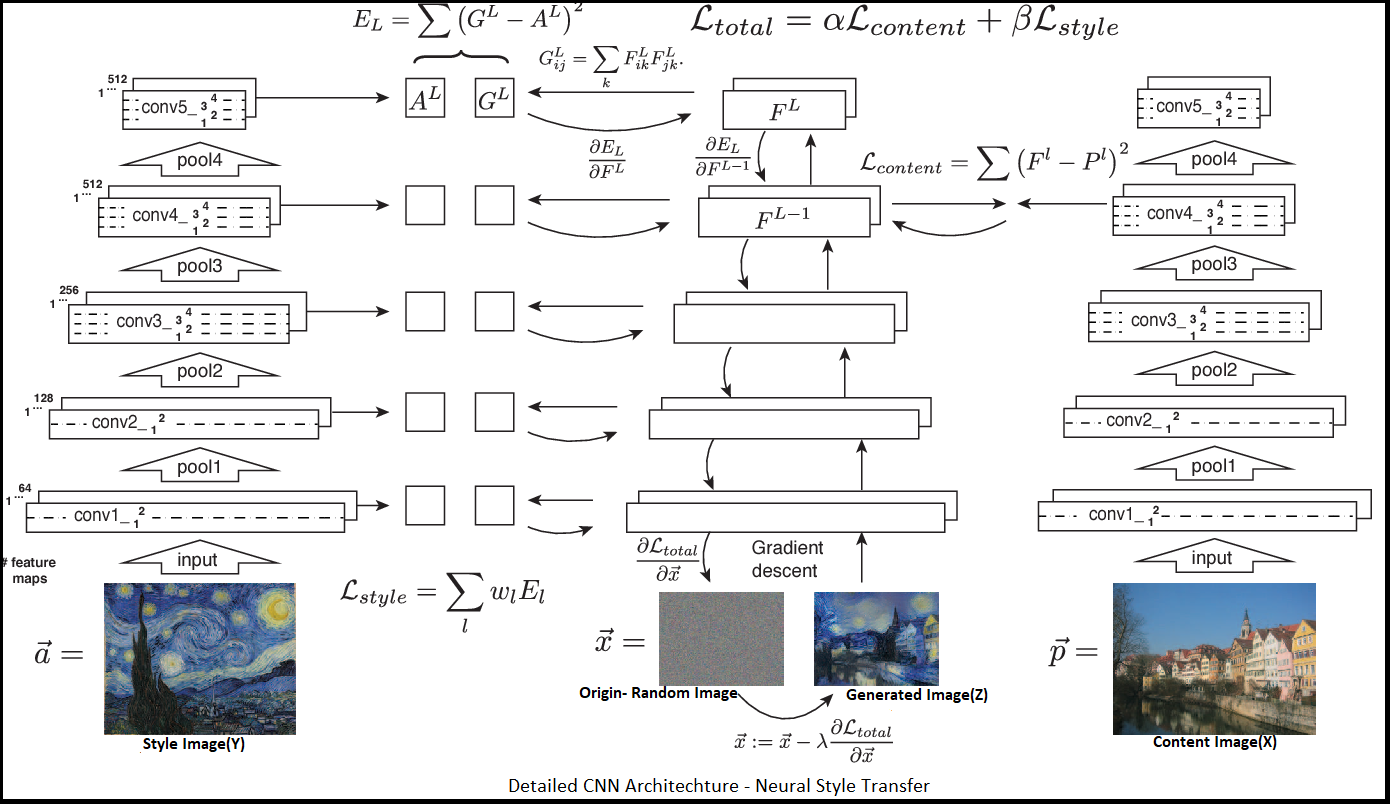So as per above typical NST network, content loss is calculated at the deeper layer to capture the high level feature(spatial), whereas for style image to capture detailed style features(texture, colours etc.) style loss is calculated by extracting network output(activation map) at shallow layer(conv-1) of each conv block. A typical pre-trained classification CNN like VGG16 is consist of a few conv blocks, which has 2 or 3 convolution(Conv2D) layers(conv1,conv2 etc.) followed by pooling(max/average) layer.So style image network is a multi-output model. In the next section, we will briefly discuss the implementation of this concept on real-time video data. The detailed code along with all inputs(content video and style image) and output(generated image frames) is found here.

### Neural Style Transfer on real-time Video:

I will explain the steps for an image, as a video is nothing but a collection of a set of images. These images are called frames and can be combined to get the original video.So we can loop through the steps for all individual frames, recombine and generate the stylized video.

Building(training) the CNN from scratch for NST application takes a lot of time and powerful computation infrastructure, which is not readily available as an individual.

So, we will load the weights(trained from images of famous ‘ImageNet.’ challenge) of a pre-trained CNN- VGG-16 to implement neural style transfer. We will use Keras Applications to load VGG-16 with pre-trained weights. VGG-16 may not optimum(desired complexity)CNN architecture for NST. There are more complex(deeper with advanced architecture) networks like InceptionV4, VGG-19,Resnet-101 etc for this application, which will take more time in loading and running. However, as an experiment, we chose a VGG-16(having high classification accuracy and good intrinsic understanding of features).

```from keras.applications.vgg16 import VGG16shape = (224,224)vgg = VGG16(input_shape=shape,weights='imagenet',include_top=False)
```

The shape is important here as the VGG-16 network takes the input image with shape 224 x 224 x 3.

```vgg.summary()_________________________________________________________________Layer (type) Output Shape Param # =================================================================input_21 (InputLayer) (None, 224, 224, 3) 0 _________________________________________________________________block1_conv1 (Conv2D) (None, 224, 224, 64) 1792 _________________________________________________________________block1_conv2 (Conv2D) (None, 224, 224, 64) 36928 _________________________________________________________________average_pooling2d_101 (Avera (None, 112, 112, 64) 0 _________________________________________________________________block2_conv1 (Conv2D) (None, 112, 112, 128) 73856 _________________________________________________________________block2_conv2 (Conv2D) (None, 112, 112, 128) 147584 _________________________________________________________________average_pooling2d_102 (Avera (None, 56, 56, 128) 0 _________________________________________________________________block3_conv1 (Conv2D) (None, 56, 56, 256) 295168 _________________________________________________________________block3_conv2 (Conv2D) (None, 56, 56, 256) 590080 _________________________________________________________________block3_conv3 (Conv2D) (None, 56, 56, 256) 590080 _________________________________________________________________average_pooling2d_103 (Avera (None, 28, 28, 256) 0 _________________________________________________________________block4_conv1 (Conv2D) (None, 28, 28, 512) 1180160 _________________________________________________________________block4_conv2 (Conv2D) (None, 28, 28, 512) 2359808 _________________________________________________________________block4_conv3 (Conv2D) (None, 28, 28, 512) 2359808 _________________________________________________________________average_pooling2d_104 (Avera (None, 14, 14, 512) 0 _________________________________________________________________block5_conv1 (Conv2D) (None, 14, 14, 512) 2359808 _________________________________________________________________block5_conv2 (Conv2D) (None, 14, 14, 512) 2359808 _________________________________________________________________block5_conv3 (Conv2D) (None, 14, 14, 512) 2359808 _________________________________________________________________average_pooling2d_105 (Avera (None, 7, 7, 512) 0 =================================================================Total params: 14,714,688Trainable params: 14,714,688Non-trainable params: 0
```

VGG-16 Architecture

Step 2: Define the content model and cost function

For high-level content features, we want to account for features across the entire image.So we will replace max-pool(which may throw away some information), with average pool.Then we will pick any deeper layer out of total 13 convolutions as the “output” and define the model up to that layer. Then we will feed our pre-processed content image(X) in the n/w to calculate(predicted) feature/activation map at the output layer wrt this model and model output wrt to any random(white noise)matrix of the defined shape(224 x 224 x 3). We calculate MSE loss and gradients for the content image network.This will help to update the input image(random image) to the opposite direction of the gradient and allow the content loss value to decrease, so that generated image will match that of the input image. The detailed implementation code is kept at my GitHub repository.

```content_model = vgg_cutoff(shape, 13) #Can be experimented with other deep layers# make the targettarget = K.variable(content_model.predict(x))# try to match the input image# define loss in kerasloss = K.mean(K.square(target - content_model.output))# gradients which are needed by the optimizergrads = K.gradients(loss, content_model.input)
```

Step 3: Define the style model and style loss function

Two images whose feature maps at a given layer produced the same Gram matrix we would expect both images to have the same style(but not necessarily the same content).So activation maps in early layers in the network would capture some of the finer textures(low level features), whereas activation maps deeper layers would capture more higher-level elements of the image’s style.So to get the best results we will take a combination of both shallow and deep layers as output to compare the style representation for an image and we define the multi-output model accordingly.

Here first we calculate Gram matrix at each layer and calculate total style loss of the style network. We take different weights for different layers to calculate the weighted loss. Then based on style loss(difference in style component) and gradients we update the input image(random image) and reducing style loss value, so that generated image(Z) texture looks similar that of the style image(Y).

`#Define multi-output modelsymb_conv_outputs = [layer.get_output_at(1) for layer in vgg.layers\if layer.name.endswith('conv1')]multi_output_model = Model(vgg.input, symb_conv_outputs)#Style feature map(outputs) of style imagesymb_layer_out = [K.variable(y) for y in multi_output_model.predect(x)]`
`#Defining Style lossdef gram_matrix(img):X = K.batch_flatten(K.permute_dimensions(img,(2,0,1)))gram_mat = K.dot(X,K.transpose(X))/img.get_shape().num_elements()return gram_mat `
`def style_loss(y,t):return K.mean(K.square(gram_matrix(y)-gram_matrix(t)))`
```#Style loss calculation through out the network#Defining layer weights for layers weights = [0.2,0.4,0.3,0.5,0.2]loss=0for symb,actual,w in zip(symb_conv_outputs,symb_layer_out,weights):loss += w * style_loss(symb,actual)grad = K.gradients(loss,multi_output_model.input)get_loss_grad = K.Function(inputs=[multi_output_model.input], outputs=[loss] + grad)
```

Step 4: Define the total cost(overall loss):

Now we can combine both content and style loss to obtain an overall loss of the network.We need to minimize this quantity over iteration using a suitable optimization algorithm.

```#Content Lossloss=K.mean(K.square(content_model.output-content_target)) * Wc #Wc is content loss weight(hyperparameter)
```
```#Defining layer weights of layers for style loss weights = [0.2,0.4,0.3,0.5,0.2]
```
```#Total loss and gradientfor symb,actual,w in zip(symb_conv_outputs,symb_layer_out,weights):loss += Ws * w * style_loss(symb,actual) #Wc is content loss weight(hyperparameter)grad = K.gradients(loss,vgg.input)get_loss_grad = K.Function(inputs=[vgg.input], outputs=[loss] + grad)
```

Step 5: Solve the optimization problem and loss minimization function

After defining the entire symbolic computation graph optimization algorithm is the principal component, which will enable to iteratively minimize the overall network cost. Here instead of using keras standard optimizer function (such as optimizers.Adam, optimizers.sgd etc.), which may take more time, we will use Limited-memory BFGS( Broyden–Fletcher–Goldfarb–Shanno), which is an approximate Numerical Optimization algorithm using a limited amount of computer memory. Due to its resulting linear memory requirement, this method is well suited for optimization problem involving large no of variables(parameters). Like normal BFGS it’s a standard quasi-Newton method to optimize smooth functions by maximizing the regularized log-likelihood.

Scipy’s minimizer function(fmin_l_bfgs_b) allows us to pass back function value f(x) and its gradient f’(x), which we calculated in earlier step. However, we need to unroll the input to minimizer function in1-D array format and both loss and gradient must be np.float64.

```#Wrapper Function to feed loss and gradient with proper format to L-BFGS def get_loss_grad_wrapper(x_vec):l,g = get_loss_grad([x_vec.reshape(*batch_shape)])return l.astype(np.float64), g.flatten().astype(np.float64)#Function to minimize loss and iteratively generate the imagedef min_loss(fn,epochs,batch_shape):t0 = datetime.now()losses = []x = np.random.randn(np.prod(batch_shape))for i in range(epochs):x, l, _ = scipy.optimize.fmin_l_bfgs_b(func=fn,x0=x,maxfun=20)# bounds=[[-127, 127]]*len(x.flatten())#x = np.clip(x, -127, 127)# print("min:", x.min(), "max:", x.max())print("iter=%s, loss=%s" % (i, l))losses.append(l)print("duration:", datetime.now() - t0)plt.plot(losses)plt.show()newimg = x.reshape(*batch_shape)final_img = unpreprocess(newimg)return final_img
```

Step 6: Run the optimizer function on input content and style image:

Run the optimizers on the input content frame and style image and as per defined symbolic computation graph, the network does its intended job of minimizing overall loss and generate an image which looks as close as to both content and style image.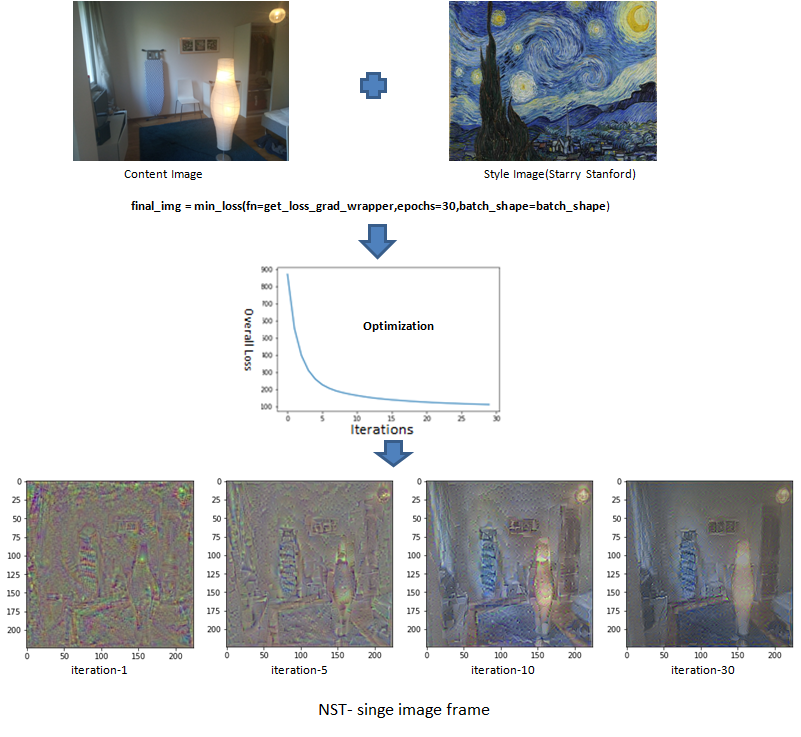The output image is still noisy, as we ran the network only for 30 iterations. The ideal NST the network should be optimized for thousands of iterations to reach the minimum loss threshold to generate clear blended output.

Step 7: Repeat the above steps for all image frames:

Perform network inference on each frame after extracting frames from the short video, generate styled image for each frame and recombine/stitch the styled image frame.

```#Vedio Reading and extracting frames
```
```cap = cv2.VideoCapture(path)while(True):ret, frame = cap.read()frame = cv2.resize(frame,(224,224))X = preprocess_img(frame)
```
``` #Running the above optimization as per defined comutation graph and generate styled image frame# final_img = min_loss(fn=get_loss_grad_wrapper,epochs=30,batch_shape=batch_shape) plt.imshow(scale(final_img))plt.show()cv2.imwrite(filename, final_img)
```
```#Recombine styled image frames to form the videovideo = cv2.VideoWriter(video_name, 0, 1, (width,height))for image in images:video.write(cv2.imread(os.path.join(image_folder, image)))cv2.destroyAllWindows()video.release()
```

We can try with videos using device camera roll as well and try the style transfer in online mode(real-time video), just by tweaking VideoCapture mode.

```cap = cv2.VideoCapture(0)cap.release()
```

Apart from personal and artistic usage of this seemingly fancy technique, Neural Style Transfer has its potential to transform any industry, where human creativity has traditionally dominated, such as fine arts, fashion, architecture or new trendy car texture design etc. For example, take the Fashion industry in which requires a deep understanding of mechanisms of fashion: causes and spreading of the trends, principles of cyclic repetition and evolution patterns to develop the fashion of tomorrow.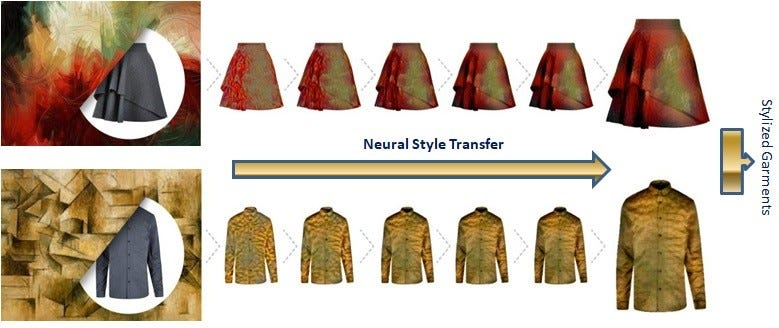Picture Courtesy

However, Neural Networks or NST can help to devise new designs by automatically allocating shapes, elements and creative textures(styles) for different types of clothing and further combine them to produce trendy fashion for tomorrow. By automating the significant portion of NST has the potential to drastically reduce the apparel design process. For further understanding, I encourage you to read this article.

### Further Improvement and Experiments:

Following are a few strategies to improve the quality of the generated image:

1) More Iterations: It is more obvious that running the network for more iterations(approximately 1000)will reduce the overall loss and will create more clear blended images.

2) Advanced CNN architecture: More often than not for NST application deeper neural networks with very advanced connection can capture high level(spatial) and detailed texture features more accurately. So it’s worth trying with other superior pre-trained networks like InceptionV4, GoogLeNet, Resnet-101 etc. However runtime of these networks is significantly high for NST application with thousands of iteration and require expensive computation infrastructure like powerful GPU stacks.

3) Tweaking content and style loss weights: As an experiment, I tried with 4 and 0.03 as content and style loss weights respectively, mainly to focus on capturing content as much as possible(as I am running the network for only a few iterations). However, this might not be appropriate and the ideal way to find the optimal weights is through a grid search

4) Tweaking layer weights for style loss: To maximize style feature capture we need to tweak weights in respective conv layers for controlling style loss computation to optimize the extraction of textures(finer textures at early layers and higher-level features at deeper layers). Again these are hyperparameters and grid search is the ideal option.

Also, we can play with the layer(L) to extract the content features from. L is also a hyperparameter of the network.

Categories Featured Excerpt